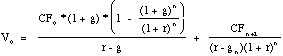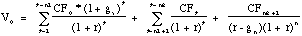DISCOUNTED CASHFLOW MODELS: WHAT THEY ARE AND HOW TO CHOOSE THE RIGHT ONE..

THE FUNDAMENTAL CHOICES FOR DCF VALUATION

• Cashflows to Discount
• Dividends
• Free Cash Flows to Equity
• Free Cash Flows to Firm
• Expected Growth
• Stable Growth
• Two Stages of Growth: High Growth -> Stable Growth
• Three Stages of Growth: High Growth -> Transition Period -> Stable Growth
• Discount Rate
• Cost of Equity
• Cost of Capital
• Base Year Numbers
• Current Earnings / Cash Flows
• Normalized Earnings / Cash Flows

WHICH CASH FLOW TO DISCOUNT...

• The Discount Rate should be consistent with the cash flow being discounted
• Cash Flow to Equity -> Cost of Equity
• Cash Flow to Firm -> Cost of Capital
• Should you discount Cash Flow to Equity or Cash Flow to Firm?
• Use Equity Valuation
• (a) for firms which have stable leverage, whether high or not, and
• (b) if equity (stock) is being valued
• Use Firm Valuation
• (a) for firms which have high leverage, and expect to lower the leverage over time, because
• debt payments do not have to be factored in
• the discount rate (cost of capital) does not change dramatically over time.
• (b) for firms for which you have partial information on leverage (eg: interest expenses are missing..)
• (c) in all other cases, where you are more interested in valuing the firm than the equity. (Value Consulting?)
• Given that you discount cash flow to equity, should you discount dividends or Free Cash Flow to Equity?
• Use the Dividend Discount Model
• (a) For firms which pay dividends (and repurchase stock) which are close to the Free Cash Flow to Equity (over a extended period)
• (b)For firms where FCFE are difficult to estimate (Example: Banks and Financial Service companies)
• Use the FCFE Model
• (a) For firms which pay dividends which are significantly higher or lower than the Free Cash Flow to Equity. (What is significant? ... As a rule of thumb, if dividends are less than 75% of FCFE or dividends are greater than FCFE)
• (b) For firms where dividends are not available (Example: Private Companies, IPOs)

WHAT IS THE RIGHT GROWTH PATTERN...

• The ChoicesTHE PRESENT VALUE FORMULAE

• For Stable Firm:• For two stage growth:• For three stage growth:Definitions of Terms

V0= Value of Equity (if cash flows to equity are discounted) or Firm (if cash flows to firm are discounted)

CFt = Cash Flow in period t; Dividends or FCFE if valuing equity or FCFF if valuing firm.

r = Cost of Equity (if discounting Dividends or FCFE) or Cost of Capital (if discounting FCFF)

g = Expected growth rate in Cash Flow being discounted

ga= Expected growth in Cash Flow being discounted in first stage of three stage growth model

gn= Expected growth in Cash Flow being discounted in stable period

n = Length of the high growth period in two-stage model

n1 = Length of the first high growth period in three-stage model

n2 - n1 = Transition period in three-stage model

WHICH MODEL SHOULD I USE?

• Use the growth model only if cash flows are positive
• Use the stable growth model, if
• the firm is growing at a rate which is below or close (within 1-2% ) to the growth rate of the economy
• Use the two-stage growth model if
• the firm is growing at a moderate rate (... within 8% of the stable growth rate)
• Use the three-stage growth model if
• the firm is growing at a high rate (... more than 8% higher than the stable growth rate)

SUMMARIZING THE MODEL CHOICES

 Dividend Discount Model FCFE Model FCFF Model Stable Growth Model Growth rate in firmís earnings is stable. (g of firmeconomy+1%) Dividends are close to FCFE (or) FCFE is difficult to compute. Leverage is stable Growth rate in firmís earnings is stable. (gfirmeconomy+1%) Dividends are very different from FCFE (or) Dividends not available (Private firm) Leverage is stable Growth rate in firmís earnings is stable. (gfirmeconomy+1%) Leverage is high and expected to change over time (unstable). Two-Stage Model Growth rate in firmís earnings is moderate. Dividends are close to FCFE (or) FCFE is difficult to compute. Leverage is stable Growth rate in firmís earnings is moderate. Dividends are very different from FCFE (or) Dividends not available (Private firm) Leverage is stable Growth rate in firmís earnings is moderate. Leverage is high and expected to change over time (unstable). Three-Stage Model Growth rate in firmís earnings is high. Dividends are close to FCFE (or) FCFE is difficult to compute. Leverage is stable Growth rate in firmís earnings is high. Dividends are very different from FCFE (or) Dividends not available (Private firm) Leverage is stable Growth rate in firmís earnings is high. Leverage is high and expected to change over time (unstable).

GROWTH AND FIRM CHARACTERISTICS

 Dividend Discount Model FCFE Discount Model FCFF Discount Model High growth firms generally Pay no or low dividends Earn high returns on projects (ROA) Have low leverage (D/E) Have high risk (high betas) Have high capital expenditures relative to depreciation. Earn high returns on projects Have low leverage Have high risk Have high capital expenditures relative to depreciation. Earn high returns on projects Have low leverage Have high risk Stable growth firms generally Pay large dividends relative to earnings (high payout) Earn moderate returns on projects (ROA is closer to market or industry average) Have higher leverage Have average risk (betas are closer to one.) narrow the difference between cap ex and depreciation. (Sometimes they offset each other) Earn moderate returns on projects (ROA is closer to market or industry average) Have higher leverage Have average risk (betas are closer to one.) narrow the difference between cap ex and depreciation. (Sometimes they offset each other) Earn moderate returns on projects (ROA is closer to market or industry average) Have higher leverage Have average risk (betas are closer to one.)

SHOULD I NORMALIZE EARNINGS?

• Why normalize earnings?
• The firm may have had an exceptionally good or bad year (which is not expected to be sustainable)
• The firm is in financial trouble, and its current earnings are below normal or negative.
• What types of firms can I normalize earnings for?
• The firms used to be financially healthy, and the current problems are viewed as temporary.
• The firm is a small upstart firm in an established industry, where the average firm is profitable.

HOW DO I NORMALIZE EARNINGS?

• If the firm is in trouble because of a recession, and its size has not changed significantly over time,
• Use average earnings over an extended time period for the firm

Normalized Earnings = Average Earnings from past period (5 or 10 years)
• If the firm is in trouble because of a recession, and its size has changed significantly over time,
• Use average Return on Equity over an extended time period for the firm

Normalized Earnings = Current Book Value of Equity * Average Return on Equity (Firm)

• If the firm is in trouble because of firm-specific factors, and the rest of the industry is healthy,
• Use average Return on Equity for comparable firms

Normalized Earnings = Current Book Value of Equity * Average Return on Equity (Comparables)